# Risk-Free Rate (rf)

Understand the Risk-Free Rate Concept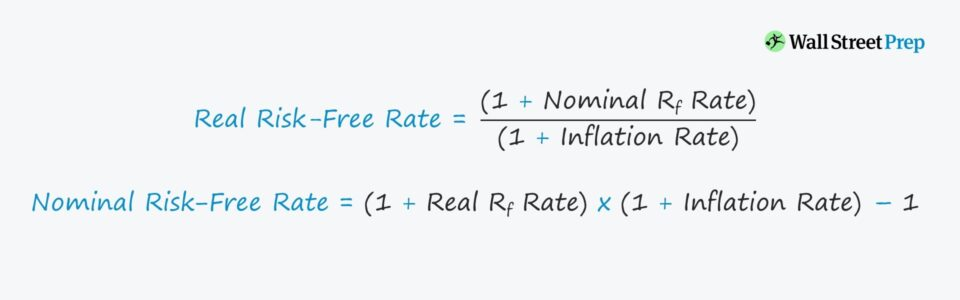• Conceptually, what does the risk-free rate represent in the context of valuation?
• What formula calculates the risk-free rate?
• What is the difference between the nominal risk-free rate and the real risk-free rate?
• How does the risk-free rate impact the cost of equity from the capital asset pricing model (CAPM)?

## Risk-Free Rate Definition

For corporate valuations, the majority of risk/return models begin with the presumption that there is a so-called “risk-free rate”.

The yield on the risk-free asset – most commonly the 10-year Treasury bond in the U.S. – is the minimum rate of return expected on investments with “zero risk” and the starting point upon which many valuation models build.

Despite the fact that the return expected by investors is considered to be risk-free, it is important to remember that the risk-free rate is a mere simplification, as all investments carry some degree of risk.

However, government-issued bonds are logically about as close to being risk-free as an asset could get, as governments could simply print more money if necessary.

As a result of being secured by a central government, the probability of default on such bond issuances is practically zero – and therefore, government bonds are viewed as the safest asset class that investors could place their capital in.

The risk-free rate should ideally match the duration of the forecast period of the cash flows, however, the limited liquidity and data for the longest maturity government-issued bonds have made the current yield on 10-year US treasury notes the preferred risk-free rate proxy in the U.S.

## Real Risk-Free Rate vs Nominal Risk-Free Rate

To expand further on the risk-free rate, there are two types to consider:

1. Real Risk-Free Rate
2. Nominal Risk-Free Rate

The reasoning behind these two concepts is related to the inclusion (or exclusion) of the rate of inflation.

The real risk-free rate is the required rate of return on zero-risk financial instruments with the rate of inflation taken into account.

The relationship between the real risk-free rate and the nominal risk-free rate is depicted by the following equation:

###### Real Risk-Free Rate Formula
• Real Risk-Free Rate = (1 + Nominal Rf Rate) / (1 + Inflation Rate)

As such, the nominal risk-free rate refers to the yield on a risk-free asset without the effect of inflation.

If the projected cash flows are discounted in nominal terms (i.e. reflects expected inflation), the discount rate used should also be nominal.

###### Nominal Risk-Free Rate Formula
• Nominal Risk-Free Rate = (1 + Real Rf Rate) * (1 + Inflation Rate) – 1

## Risk-Free Rate Component in CAPM

The risk-free rate has a significant role in the capital asset pricing model (CAPM), which is the most widely used model for estimating the cost of equity.

Under the CAPM, the expected return on a risky asset is estimated as the risk-free rate plus an approximated equity risk premium. The minimum returns threshold factors in the beta of the specific asset (i.e. systematic, non-diversifiable risk) and the average return of the stock market.

The risk-free rate serves as the minimum rate of return, to which the excess return (i.e. the beta multiplied by the equity risk premium) is added.

The equity risk premium (ERP) is calculated as the average market return (S&P 500) minus the risk-free rate.

###### Equity Risk Premium (ERP) Formula
• Equity Risk Premium (ERP) = Expected Market Return – Risk-Free Rate

The equity risk premium helps investors evaluate potential investment based on the “extra” return that they are receiving for the incremental risk above the risk-free rate.

In effect, the risk-free rate has broad implications on how investors allocate their capital based on prevailing market conditions.

## WACC and Risk-Free Rate

The risk-free rate assumption is also a key input in the estimation of the weighted average cost of capital (WACC) of a company.

The CAPM estimates the cost of equity based on the risk-free rate of return and the additional risk (and required return) associated with the investment.

But the cost of debt can also be estimated by adding a certain spread based on the risk profile (i.e. default risk premium) of the company to the risk-free rate.

Therefore, if the risk-free rate increases, there will be increased pressure on the equity risk premium to compensate investors more for the amount of risk undertaken (and vice versa).

Since investors can receive higher returns from risk-free assets, riskier assets are expected to result in higher returns to meet the new standards set by the market for the returns of riskier assets.

All else being equal, lower risk-free rates result in lower discount rates, which directly causes higher valuations of equities.

###### COVID Market Rebound

There are various theories as to why the markets rebounded rather quickly after bottoming around March 2020, but one reason behind the rapid recovery is the role of the risk-free rate.

Around this particular time when the market declined significantly due to the uncertainty surrounding COVID, the U.S. 10-year treasury notes ranged around 0.6% to 0.8%.

Institutional and retail investors were left with two choices:

1. Invest in risk-free government bonds yielding practically nothing (<1.0%) – with the Fed indicating no plans for interest rate hikes until the economy reached full recovery.
2. Invest in the largest, market-leading companies, most notably the “FANG” stocks (Facebook, Amazon, Apple, Netflix, and Google), which each had a significant likelihood of enduring through this period – if not even benefiting from the lock-down period.

The performance of the stock market makes it clear which decision that many investors chose, especially for the tech giants that saw a disproportionate outperformance relative to the broader market as they led the market’s swift recovery (and the eventual surpassing of record all-time highs).

## “Normalized” Risk-Free Rate

If the prevailing interest rates in the market are at historically low levels, DCF-derived valuations tend to be higher since the discount rate is going to be lower from the decline in the risk-free rate.

There has been much debate on normalized risk-free rates, with Duff & Phelps regularly publishing reports on their recommended equity risk premium (ERP), which uses a normalized version of the risk-free rate.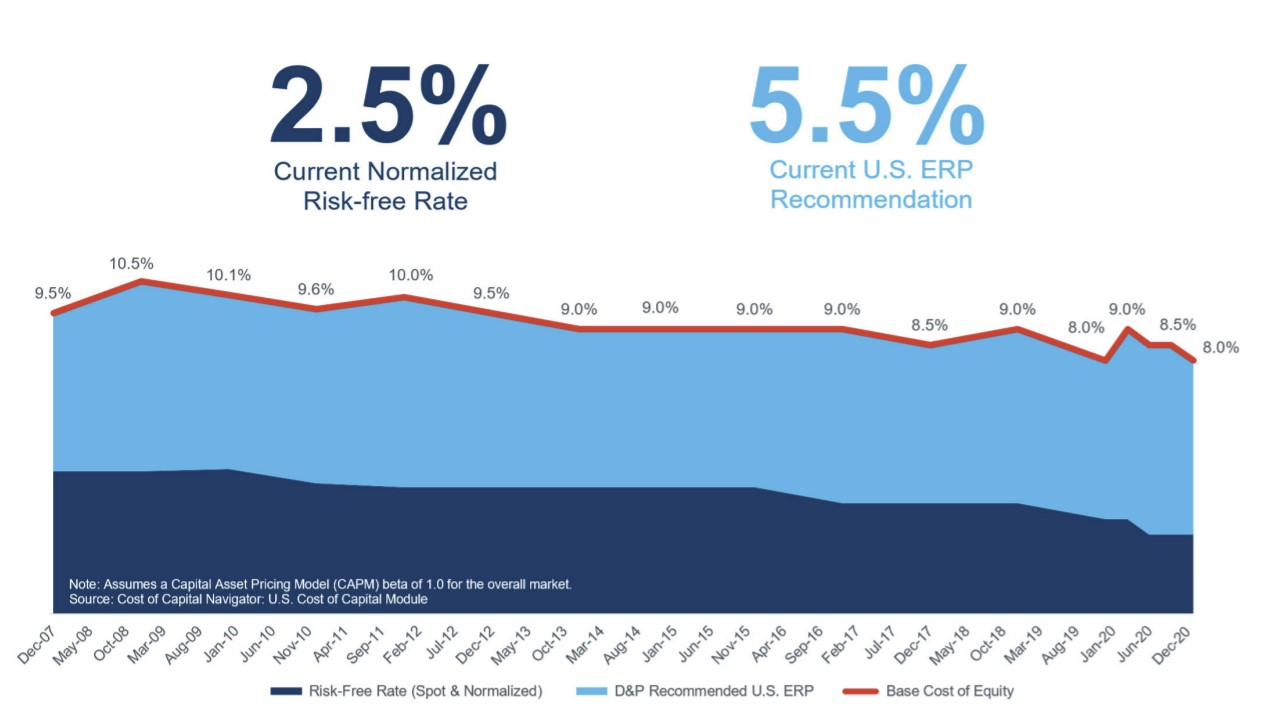Duff & Phelps Recommended Equity Risk Premium (Source: Duff & Phelps)

Conversely, NYU Professor Aswath Damodaran has argued against the use of normalized rates, stating that the risk-free rate should reflect the real opportunity cost – i.e. the actual options available – as of the present date.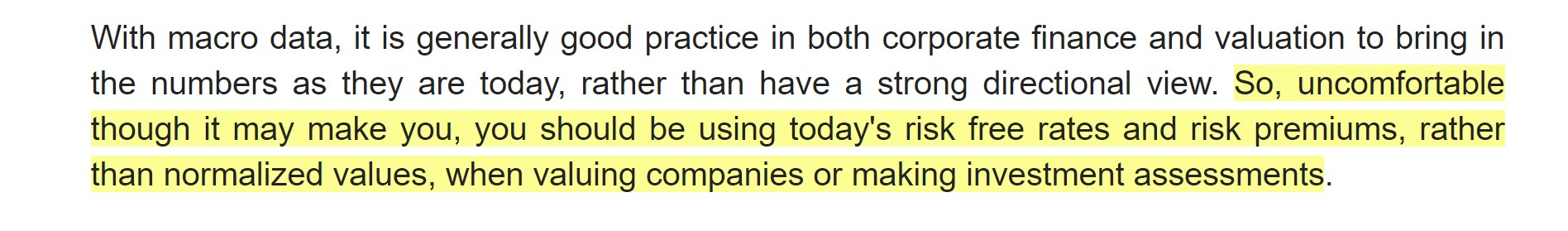Damodaran on Normalized Risk-Free Rates (Source: Damodaran – Musings on Markets)

###### LIBOR Transition

LIBOR, the standard benchmark used in the financial markets for setting interest rates on loans and various debt instruments, is being phased out by the end of 2021.

## Risk-Free Rate Calculator – Excel Template

We’ll now move to a modeling exercise, which you can access by filling out the form below.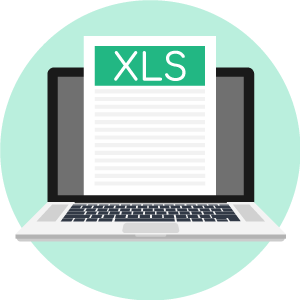Submitting ...

## Risk-Free Rate Example Calculation

For our risk-free rate modeling exercise, we’ll first calculate the nominal risk-free rate and then move to the real risk-free rate.

###### Model Assumptions
• Real Risk-Free Rate = 5.0%
• Inflation Rate = 3.0%

From those two assumptions, we’ll enter them into the formula to calculate the nominal risk-free rate:

• Nominal Risk-Free Rate = (1 + Real Risk-Free Rate) * (1 + Inflation Rate) – 1
• Nominal Risk-Free Rate = (1 + 5.0%) * (1 + 3.0%) – 1

Here, the nominal risk-free rate comes out to 8.2%.

Next, we’ll calculate the real risk-free rate using the same assumptions.

• Real Risk-Free Rate = (1 + Nominal Risk-Free Rate) / (1 + Inflation Rate) – 1
• Real Risk-Free Rate = (1 + 8.2%) / (1 + 3.0%) – 1

As expected, we arrive at 5.0% for our real risk-free rate, which is the rate of return on the risk-free security once adjusted for the impact of inflation.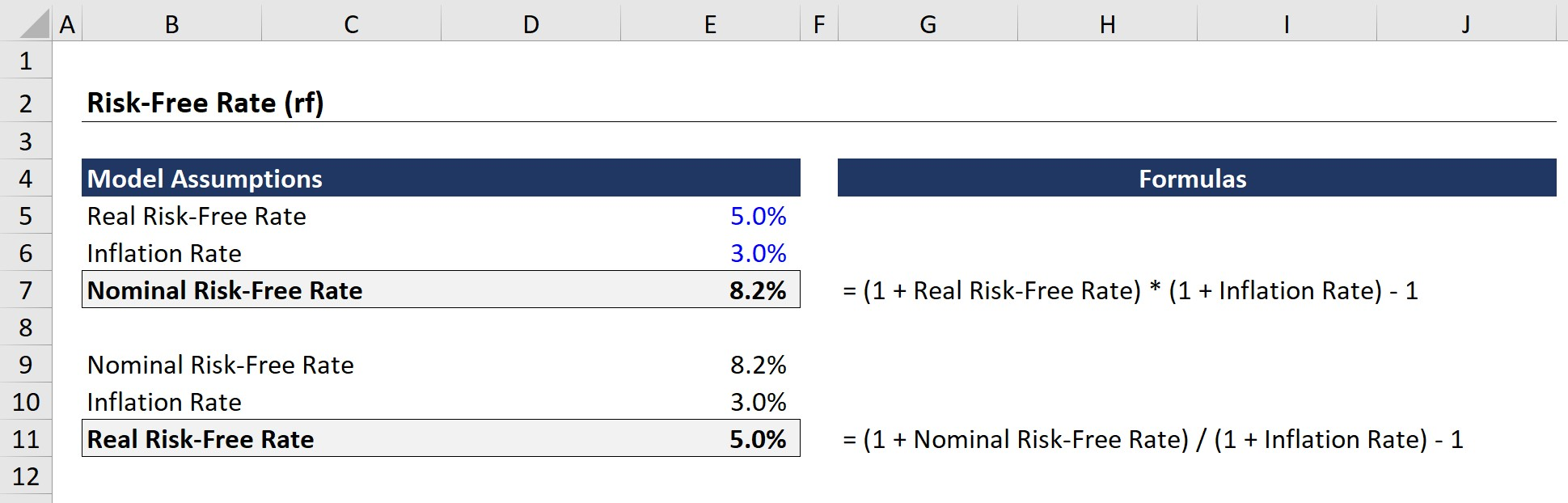Step-by-Step Online Course

#### Everything You Need To Master Financial Modeling

Enroll in The Premium Package: Learn Financial Statement Modeling, DCF, M&A, LBO and Comps. The same training program used at top investment banks.Inline FeedbacksLearn Financial Modeling Online

Everything you need to master financial and valuation modeling: 3-Statement Modeling, DCF, Comps, M&A and LBO.

X

The Wall Street Prep Quicklesson Series

7 Free Financial Modeling Lessons

Get instant access to video lessons taught by experienced investment bankers. Learn financial statement modeling, DCF, M&A, LBO, Comps and Excel shortcuts.